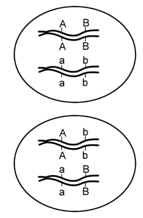# 24.5: Inferring Recombination From Genetic Data

$$\newcommand{\vecs}{\overset { \rightharpoonup} {\mathbf{#1}} }$$ $$\newcommand{\vecd}{\overset{-\!-\!\rightharpoonup}{\vphantom{a}\smash {#1}}}$$$$\newcommand{\id}{\mathrm{id}}$$ $$\newcommand{\Span}{\mathrm{span}}$$ $$\newcommand{\kernel}{\mathrm{null}\,}$$ $$\newcommand{\range}{\mathrm{range}\,}$$ $$\newcommand{\RealPart}{\mathrm{Re}}$$ $$\newcommand{\ImaginaryPart}{\mathrm{Im}}$$ $$\newcommand{\Argument}{\mathrm{Arg}}$$ $$\newcommand{\norm}{\| #1 \|}$$ $$\newcommand{\inner}{\langle #1, #2 \rangle}$$ $$\newcommand{\Span}{\mathrm{span}}$$ $$\newcommand{\id}{\mathrm{id}}$$ $$\newcommand{\Span}{\mathrm{span}}$$ $$\newcommand{\kernel}{\mathrm{null}\,}$$ $$\newcommand{\range}{\mathrm{range}\,}$$ $$\newcommand{\RealPart}{\mathrm{Re}}$$ $$\newcommand{\ImaginaryPart}{\mathrm{Im}}$$ $$\newcommand{\Argument}{\mathrm{Arg}}$$ $$\newcommand{\norm}{\| #1 \|}$$ $$\newcommand{\inner}{\langle #1, #2 \rangle}$$ $$\newcommand{\Span}{\mathrm{span}}$$

In the preceding examples, we had the advantage of knowing the approximate chromosomal positions of each allele involved, before we calculated the recombination frequencies. Knowing this information beforehand made it relatively easy to define the parental and recombinant genotypes, and to calculate recombination frequencies. However, in most experiments, we cannot directly examine the chromosomes, or even the gametes, so we must infer the arrangement of alleles from the phenotypes over two or more generations. Importantly, it is generally not sufficient to know the genotype of individuals in just one generation; for example, given an individual with the genotype AaBb, we do not know from the genotype alone whether the loci are located on the same chromosome, and if so, whether the arrangement of alleles on each chromosome is AB and ab or Ab and aB (Figure $$\PageIndex{6}$$). The top cell has the two dominant alleles together and the two recessive alleles together and is said to have the genes in the coupling (or cis) configuration. The alternative shown in the cell below is that the genes are in the repulsion (or trans) configuration.Figure $$\PageIndex{6}$$: Alleles in coupling configuration (top) or repulsion configuration (bottom). (Original-Deyholos-CC:AN)

Fortunately for geneticists, the arrangement of alleles can sometimes be inferred if the genotypes of a previous generation are known. For example, if the parents of AaBb had genotypes AABB and aabb respectively, then the parental gametes that fused to produce AaBb would have been genotype AB and genotype ab. Therefore, prior to meiosis in the dihybrid, the arrangement of alleles would likewise be AB and ab (Figure $$\PageIndex{7}$$). Conversely, if the parents of AaBb had genotypes aaBB and AAbb, then the arrangement of alleles on the chromosomes of the dihybrid would be aB and Ab. Thus, the genotype of the previous generation can determine which of an individual’s gametes are considered recombinant, and which are considered parental.Figure $$\PageIndex{7}$$: The genotype of gametes can be inferred unambiguously if the gametes are produced by homozygotes. However, recombination frequencies can only be measured among the progeny of heterozygotes (i.e. dihybrids). Note that the dihybrid on the left contains a different configuration of alleles than the dihybrid on the rightdue to differences in the genotypes of their respective parents. Therefore, different gametes are defined as recombinant and parental among the progeny of the two dihybrids. In the cross at left, the recombinant gametes will be genotype AB and ab, and in the cross on the right, the recombinant gametes will be Ab and aB. (Original-Deyholos-CC:AN)

Let us now consider a complete experiment in which our objective is to measure recombination frequency (Figure $$\PageIndex{8}$$). We need at least two alleles for each of two genes, and we must know which combinations of alleles were present in the parental gametes. The simplest way to do this is to start with pure-breeding lines that have contrasting alleles at two loci. For example, we could cross short-tailed mice, brown mice (aaBB) with long-tailed, white mice (AAbb). Based on the genotypes of the parents, we know that the parental gametes will be aB or Ab (but not ab or AB), and all of the progeny will be dihybrids, AaBb. We do not know at this point whether the two loci are on different pairs of homologous chromosomes, or whether they are on the same chromosome, and if so, how close together they are.Figure $$\PageIndex{8}$$: An experiment to measure recombination frequency between two loci. The loci affect coat color (B/b) and tail length (A/a). (Wikipedia-Modified Deyholos-CC:AN)

The recombination events that may be detected will occur during meiosis in the dihybrid individual. If the loci are completely or partially linked, then prior to meiosis, alleles aB will be located on one chromosome, and alleles Ab will be on the other chromosome (based on our knowledge of the genotypes of the gametes that produced the dihybrid). Thus, recombinant gametes produced by the dihybrid will have the genotypes ab or AB, and non-recombinant (i.e. parental) gametes will have the genotypes aB or Ab.

How do we determine the genotype of the gametes produced by the dihybrid individual? The most practical method is to use a testcross (Figure $$\PageIndex{8}$$), in other words to mate AaBb to an individual that has only recessive alleles at both loci (aabb). This will give a different phenotype in the F2 generation for each of the four possible combinations of alleles in the gametes of the dihybrid. We can then infer unambiguously the genotype of the gametes produced by the dihybrid individual, and therefore calculate the recombination frequency between these two loci. For example, if only two phenotypic classes were observed in the F2 (i.e. short tails and brown fur (aaBb), and white fur with long tails (Aabb) we would know that the only gametes produced following meiosis of the dihybrid individual were of the parental type: aB and Ab, and the recombination frequency would therefore be 0%. Alternatively, we may observe multiple classes of phenotypes in the F2 in ratios such as shown in Table $$\PageIndex{1}$$:

 tail phenotype fur phenotype number of progeny gamete from dihybrid genotype of F2 from test cross (P)arental or (R)ecombinant short brown 48 aB aaBb P long white 42 Ab Aabb P short white 13 ab aabb R long brown 17 AB AaBb R

Given the data in Table $$\PageIndex{1}$$, the calculation of recombination frequency is straightforward:

\begin{align} \textrm{recombination frequency} &= \mathrm{\dfrac{number\: of\: recombinant\: gametes}{total\: number\: of\: gametes\: scored}}\\ \textrm{R.F.} &= \dfrac{13+17}{48+42+13+17}\\ &=25\% \end{align}

24.5: Inferring Recombination From Genetic Data is shared under a CC BY-SA 3.0 license and was authored, remixed, and/or curated by Todd Nickle and Isabelle Barrette-Ng via source content that was edited to conform to the style and standards of the LibreTexts platform; a detailed edit history is available upon request.